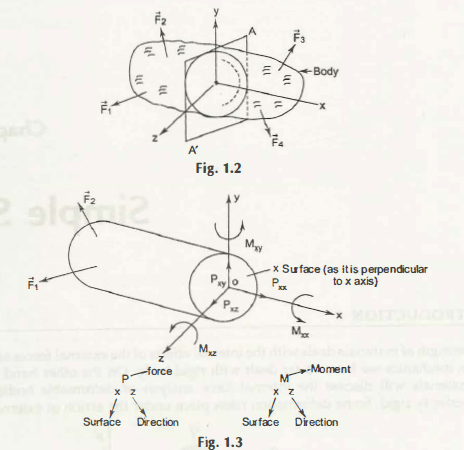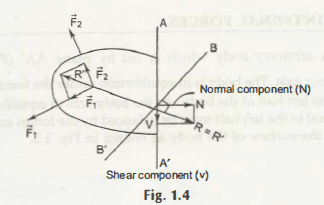Now, taking the proper decision of the type of force is somehow confused and thus it is tensile if it pulls and in case of pressing it is compressive force. Elongation of members can be seen when the force is a tensile force, however the compressive force can shortens it.

P xy’ P xz = Shear forces, these are acted parallel to the surface x. Resistance is given by it against sliding.

V is used to denote the resultant shear and in the direction of x it is Vx and in the direction of y it is Vy.

What are the different factors here those affecting “analysis of forces”?

These are –

Mu – This is a torque and provides resistance opposite to twisting member. This can be denoted by T.

Mxz is the bending moments and it also provides the resistance, but against the member’s bending.

To understand the exact way, the following figure is important to explain and here you will get that R is perpendicular to BB’, then all normal forces are generated.If there is an external force acts on the plane xy, then the three different components due to this internal effect will be –

• Pxx (or P)
• Shear force P xy (or V)
• Bending moment Mxz (or M).

Links of Next Mechanical Engineering Topics:-### Customer Reviews

My Homework Help
Rated 5.0 out of 5 based on 510 customer reviews at